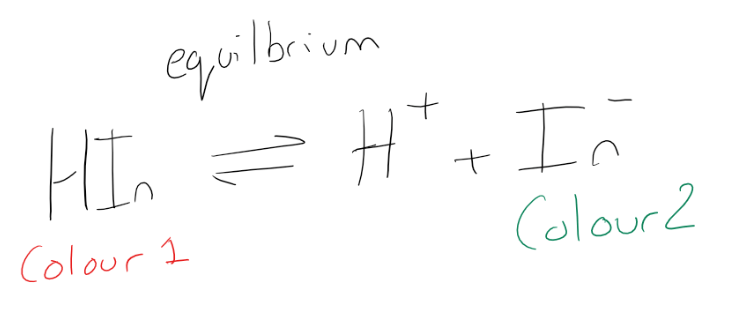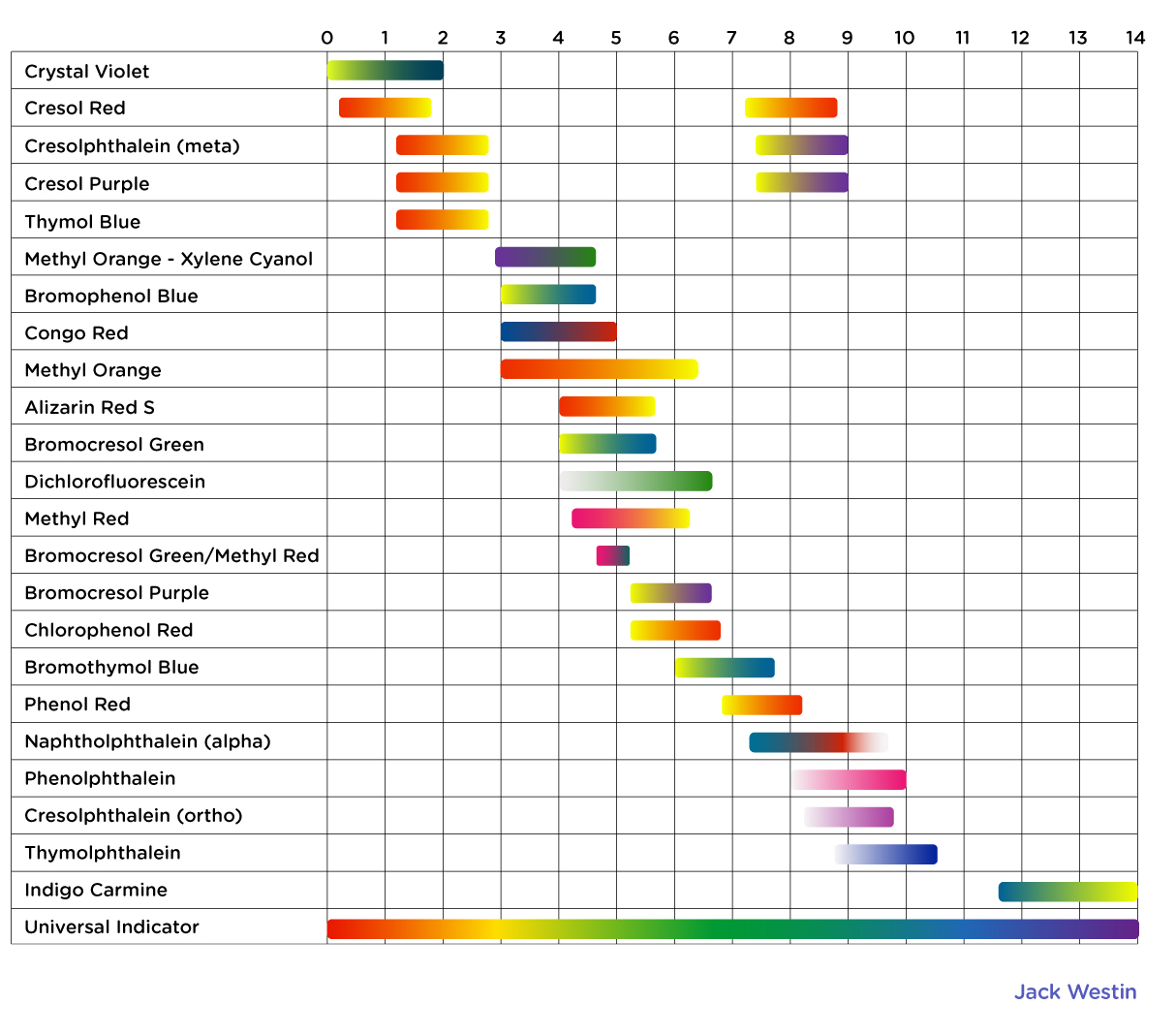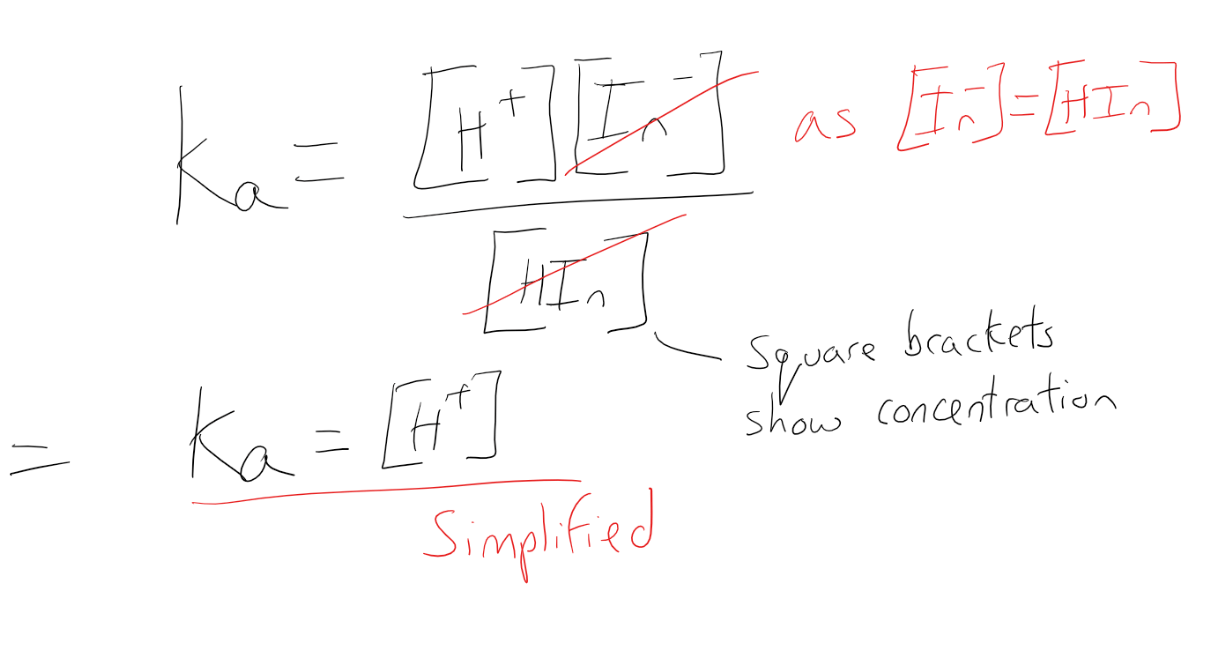Complimentary 1-hour tutoring consultation

MCAT Content / Titration / Indicators

### Indicators

Topic: Titration

An acid-base indicator is a substance that displays different colors when in the presence of an acid or a base.

An indicator is a weak acid that ionizes within a known pH range, usually about 2 pH units. We can represent the protonated form of the indicator molecule as HIn and the deprotonated form as In. The following equilibrium exists for the indicator:An indicator is a weak acid or a weak base that has different colors in its dissociated and undissociated states. An indicator reacts with an acid or base to show an equivalence point in a titration.

An indicator’s color depends on the pH of a solution. Indicators themselves are weak acids or weak bases that exist in an equilibrium between dissociated (H+ + In) and undissociated states (HIn), with each state being a different color. The equilibrium will respond to a change in the environment when H+ ions or OH ions are added

According to LeChâtelier’s principle, the addition of Hions (as in a low pH solution) drives the equilibrium to the left and the protonated HIn predominates. The addition of OH− (as in a high pH solution) decreases the Hconcentration and drives the equilibrium to the right and the deprotonated In− predominates. To be useful as an indicator, the two forms must be different colors.Depending on the pH at the equivalence point, the appropriate indicator must be chosen. For example, bromphenol blue has a yellow color below a pH of about 3 and a blue-violet color above a pH of about 4. Bromphenol blue would not be a good choice as the indicator for a strong acid-strong base titration, because the pH is 7 at the equivalence point. Instead, it could be used for a strong acid-weak base titration, where the pH at the equivalence point is lower. Most indicators have two colored forms. Universal indicator displays the entire rainbow of colors from low pH to high pH. Universal indicator is used to make pH paper, which can be used to quickly test solutions for their approximate pH.The color change that occurs in indicators is known as the endpoint and is over a range. This, however, is not the same as the equivalence point. The pH at which a color change occurs can be determined by using the acid dissociation constant Ka. Using the equilibrium of the indicator:At the point where equilibrium is balanced between the acid and the conjugate base where [In] = [HIn]. this is when the indicator is in the middle of its color change so we can simplify the equation to: Ka = [H+].

Practice Questions

MCAT Official Prep (AAMC)

Chemistry Online Flashcards Question 4

Official Guide C/P Section Passage 5 Question 24

Key Points

• In general, a molecule that changes color with the pH of the environment it is in can be used as an indicator.

• In the reaction HIn ⇌H++In, adding acid shifts the indicator equilibrium to the left.

• In the reaction HIn ⇌H++In, adding base shifts the indicator equilibrium to the right.

• The equivalence point is not the same as the endpoint (a range over which the indicator will change color).

• For optimal accuracy, the color difference between the two colored species should be as clear as possible, and the narrower the pH range of the color change the better.

Key Terms

pH: The negative of the logarithm to base 10 of the concentration of hydrogen ions, measured in moles per liter; a measure of acidity or alkalinity of a substance, which takes numerical values from 0 (maximum acidity) through 7 (neutral) to 14 (maximum alkalinity).

Equivalence point: The point at which the equivalent number of moles of a base has been added to an acid.

Endpoint: The term given to the color change of an indicator when an acid and base react

Indicator: A halochromic chemical compound that is added in small amounts to a solution so that the pH (acidity or basicity) of the solution can be determined visually.

pH indicator: An acid-base indicator.

Titration: The determination of the concentration of some substance in a solution by slowly adding measured amounts of some other substance (normally using a burette) until a reaction is shown to be complete—for instance, by the color change of an indicator.

Ka: Acid dissociation constant, a quantitative measure of the strength of an acid in solution.

LeChâtelier’s principle: If a dynamic equilibrium is disturbed by changing the conditions, the position of equilibrium shifts to counteract the change to reestablish an equilibrium.

Billing Information